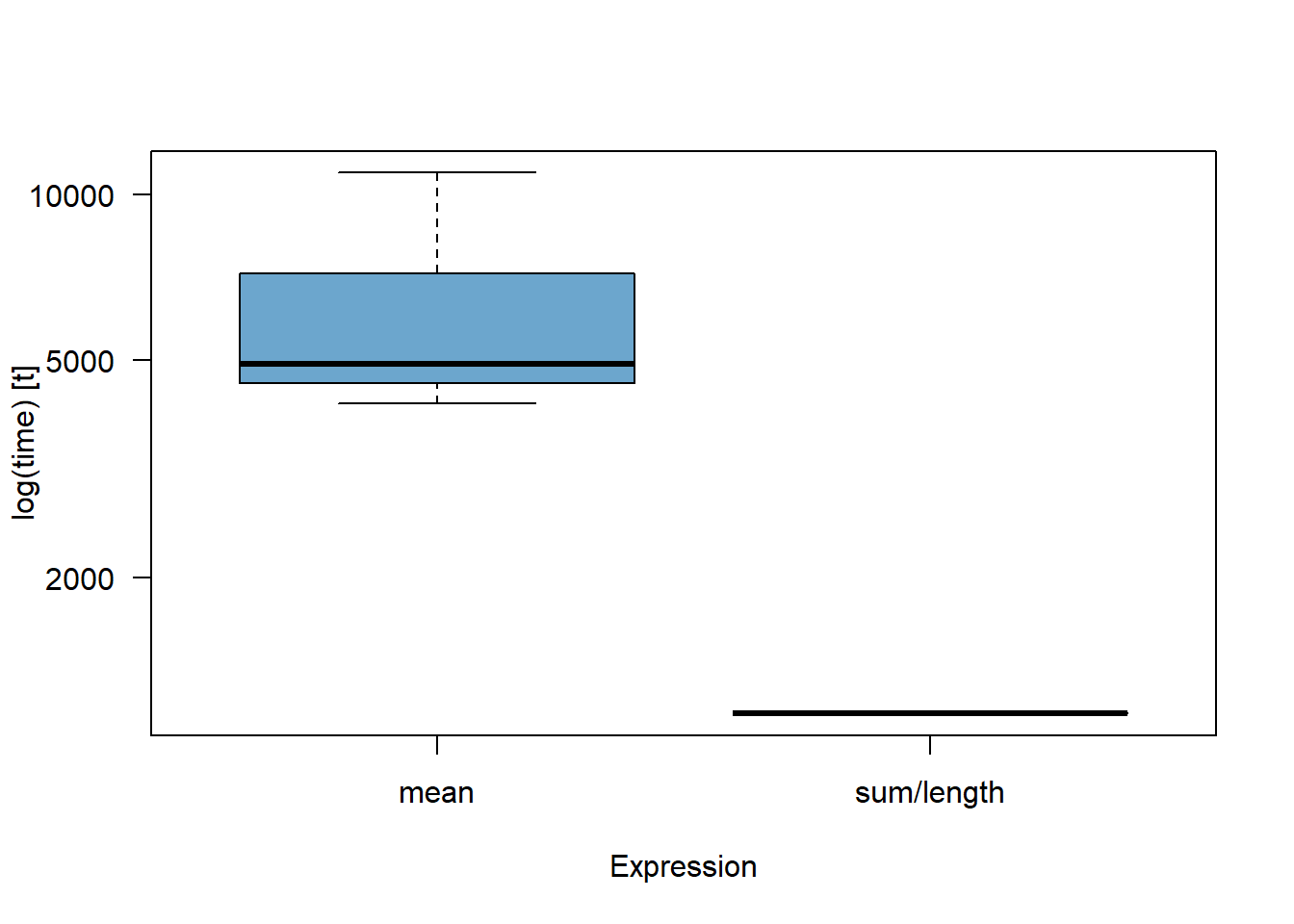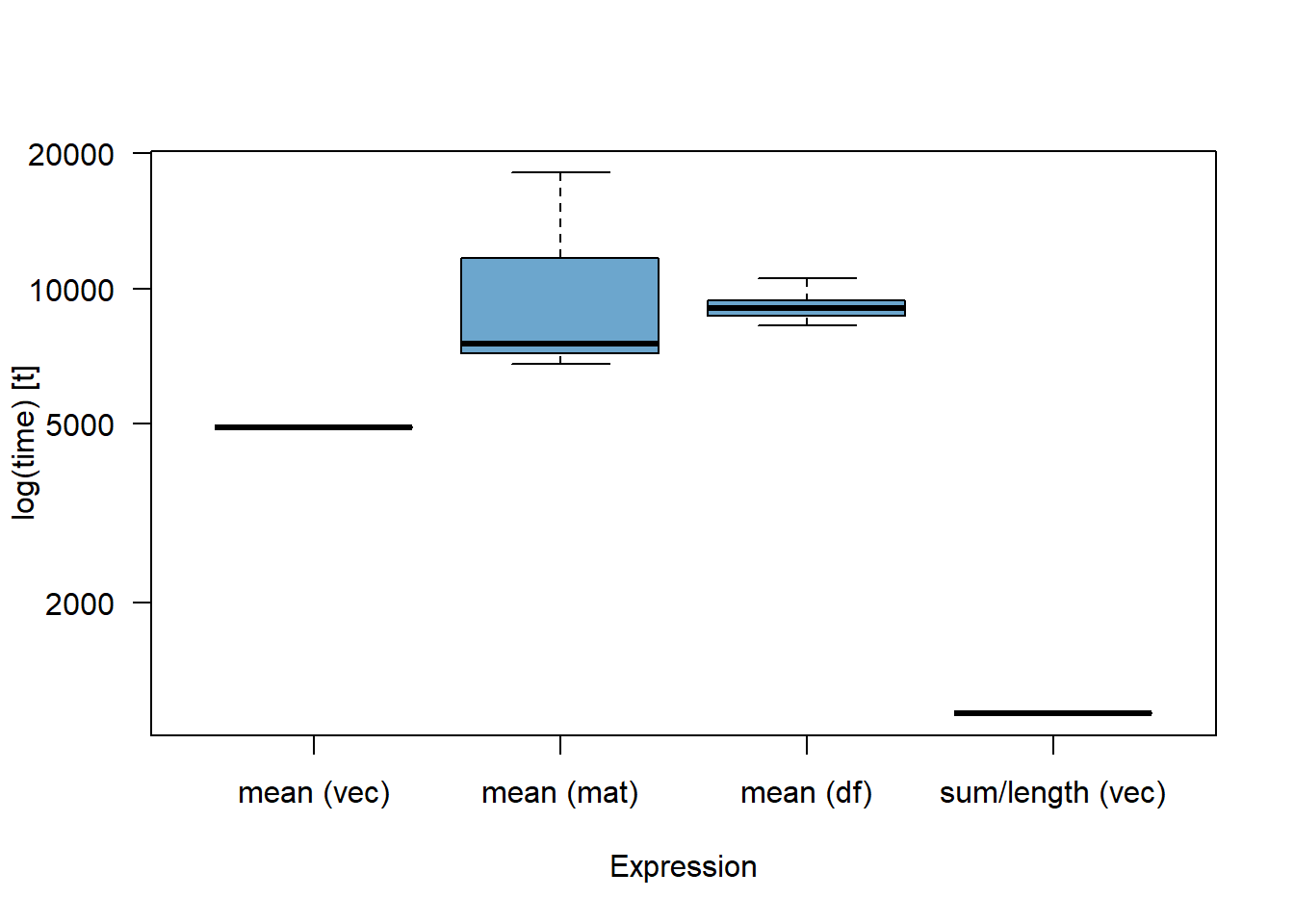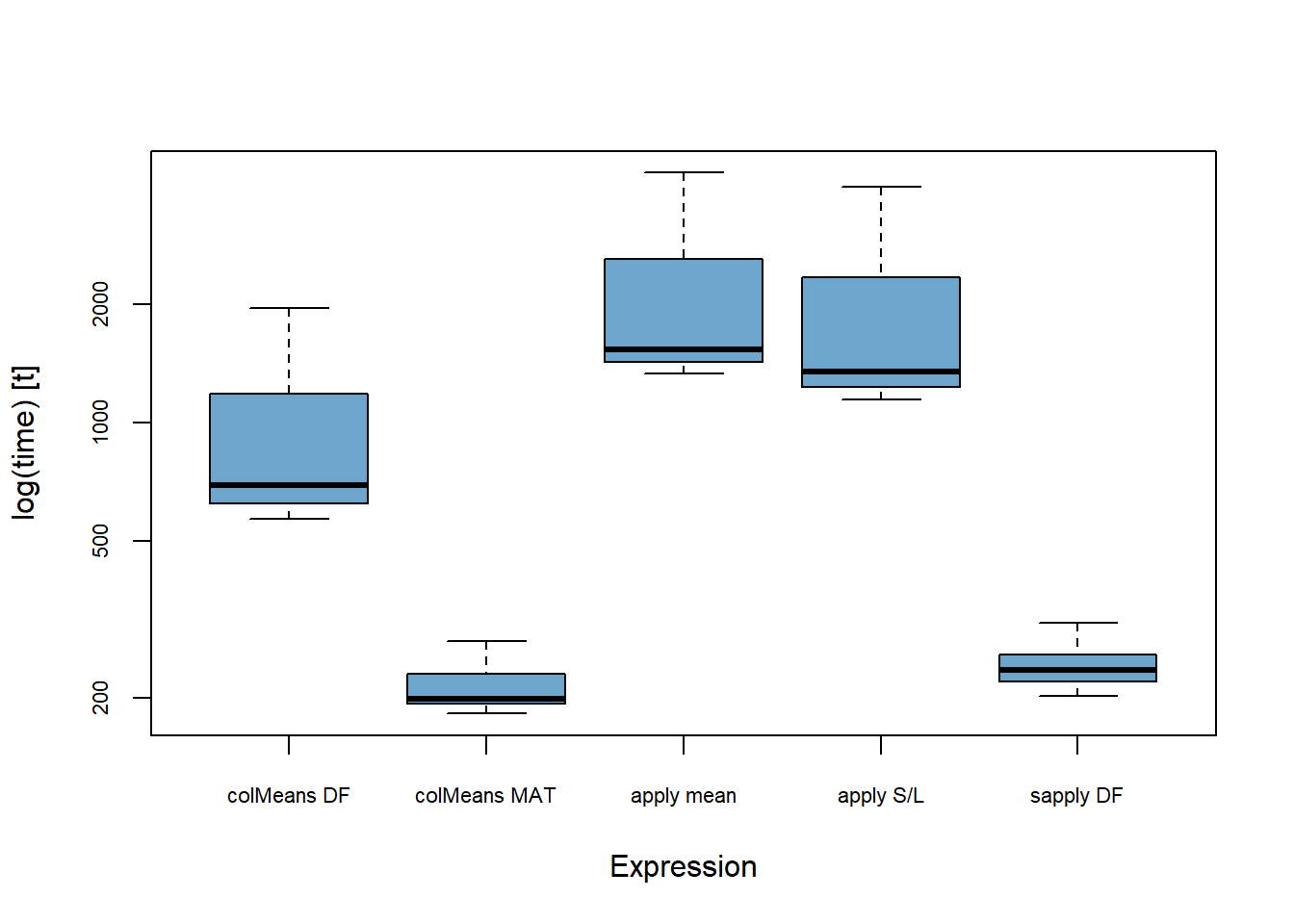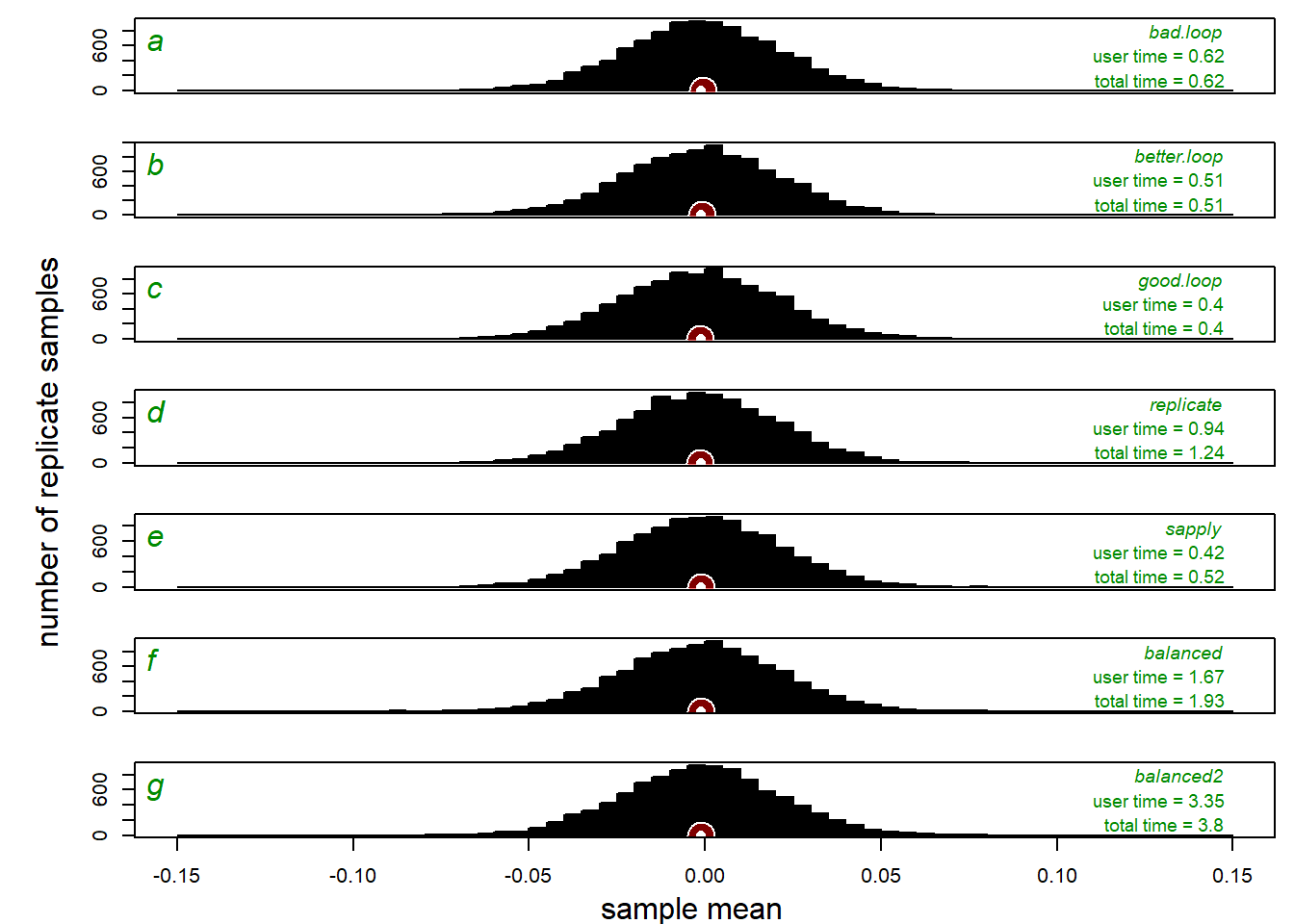## Introduction

Even for simple programming tasks, multiple solutions exist. However, while those solutions may produce identical outputs, they may differ in computational speed, flexibility, and transparency. Here, the focus will be on issues that affect computational speed (i.e., CPU usage). When datasets are small or object manipulations simple, CPU usage is not that critical. However, for massive datasets or problems that involve many iterative operations, computational efficiency becomes important. The efficiency of a programming statement is affected, among others, by functions used, types of objects, and overall script design. Let’s examine these issues using simple examples.

## FAST AND SLOW FUNCTIONS

Even for simplest operations, such as computing a univariate mean of a single set of values, different ways are available. For example, the generic function {mean} is the most commonly seen solution. However, you can also compute means by combining sum and length functions. Which of those two expressions is faster? An intuitive answer would be, perhaps, that a single function “mean” should be faster than an operation that involves two functions “sum” and “length”. However, the function “mean” is internally more complex than either “sum” or “length”, and thus, may be actually slower. Let’s investigate this more rigorously.

First, upload the package ‘microbenchmark’ (Mersmann, 2015)

library(microbenchmark)     # install this package first (Mersmann, 2015)

‘Mean’ versus ‘sum/length’

Now let’s check this issue empirically using a random sample of 1000 observations drawn from a normal distribution with mean=5 and standard deviation=1.

x1 <- rnorm(1000, mean=5)   # let's create a large sample from normal distribution
mean(x1)                # check the observed mean using {mean} function 
##  4.999321
sum(x1)/length(x1)      # compute the same value using (sum}/{length} combination
##  4.999321
# check which of the two methods for computing mean is faster
(out1 <- microbenchmark(mean(x1), sum(x1)/length(x1), times=10000)) #add( )around it to print it
## Unit: nanoseconds
##                expr  min   lq     mean median   uq   max neval
##            mean(x1) 4154 4532 7025.437   4909 7175 86844 10000
##  sum(x1)/length(x1)  755 1133 1594.014   1133 1134 35116 10000
# visualize these results
boxplot(out1, outline=F, las=1, col='skyblue3',
names=c('mean', 'sum/length'))Figure 1.Boxplot comparing estimates of computational speed (CPU usage in nanoseconds) for calculating univariate mean using two different functions (‘mean’ and ‘sum/length’). Based on 10000 trials using function “microbenchmark” in a package (microbenchmark} (Mersmann, 2015).

As is clear in this example (Fig. 1), using “sum/length” to compute mean of 1000 observations took much less time than using “mean”. This difference is an estimate specific to a vector of 1000 continuous values and values are also dependent on the computer used. The “sum/length” may be faster than “mean”, but the difference may not always be by the same factor. This is because computational speed may also vary depending on the type of the objects, object dimensions, and overall design of a given script.

## OBJECT TYPES AND VECTORIZATION

Objects stored in simple formats (vectors) tend to require less CPU time than more structured objects (matrices, lists, and data frames). Thus, we can often make our script notably faster by ensuring that time-consuming tasks are performed using vectors. By “vectorizing” your objects you can thus save time needed to perform massive data manipulations. Let’s build on an example above, and store x1 (a vector object used in Example 1 above) in other more structured, but likely less CPU-efficient formats.

x2 <- matrix(x1)            # store x1 (a vector) as a 1-column matrix
x3 <- data.frame(x1)        # store x1 (a vector) as a dataframe
# obviously, the format in which x1 is stored doesn't affect the value of the mean
c(mean(x1), mean(x2[,1]), mean(x3[]), sum(x1)/length(x1))    
##  4.999321 4.999321 4.999321 4.999321
# let's check which of the above computations is fastest
(out2 <- microbenchmark(times=10000, mean(x1), mean(x2[,1]),
mean(x3[]), sum(x1)/length(x1)))
## Unit: nanoseconds
##                expr  min   lq      mean median    uq     max neval
##            mean(x1) 4531 4909  6171.081   4909  4910 1918867 10000
##       mean(x2[, 1]) 6796 7175 10428.462   7552 11705   66455 10000
##       mean(x3[]) 8307 8685 11354.404   9063  9440 1677971 10000
##  sum(x1)/length(x1)  755 1133  1759.732   1133  1134 2903221 10000
# visualize these results
boxplot(out2, outline=F, las=1, col='skyblue3',
names=c('mean (vec)', 'mean (mat)', 'mean (df)', 'sum/length (vec)'))FIGURE 2 - Boxplot comparing estimates of computational speed (CPU usage in nanoseconds) for calculating univariate mean using different function-object combinations. Based on 10000 trials using function “microbenchmark” from a package (microbenchmark} (Mersmann, 2015). Abbreviations: mean (vec) - applying function mean to a vector x1; mean (mat) - applying function mean to a matrix x2 with a single column containing vector x1; mean (df) - applying function “mean” to a data frame x3 that represents a single list containing vector x1; sum/length (vec) - applying functions “sum” and “length” to a vector x1.

Note (Fig. 2) that when mean is applied to a vector, the operation is notably faster comparing to matrix and data frame. If we use sum/length combination and apply it to a vector (the fastest option here), our CPU time drops notably relative to using function ‘mean’ applied to a column from a matrix or a list from a dataframe.

y1 <- data.frame(rnorm(100000,10,1), rnorm(100000,20,2))
y2 <- cbind(rnorm(100000,10,1), rnorm(100000,20,2))
out3 <- microbenchmark(colMeans(y1), colMeans(y2), apply(y2, 2, mean),
apply(y2, 2, function(x) sum(x)/length(x)),
sapply(y1, function(x) sum(x)/length(x)))
out3p <- summary(out3)
out3p[-c(3,5,6,8)]
##                                         expr      min      mean      max
## 1                               colMeans(y1)  569.015  957.9275 4299.894
## 2                               colMeans(y2)  182.750  223.9628  551.646
## 3                         apply(y2, 2, mean) 1332.862 2150.8268 8411.751
## 4 apply(y2, 2, function(x) sum(x)/length(x)) 1141.051 1903.8775 6816.849
## 5   sapply(y1, function(x) sum(x)/length(x))  202.006  264.3677 1511.457
boxplot(out3, cex.axis=0.7, outline=F, col='skyblue3',
names=c('colMeans DF', 'colMeans MAT', 'apply mean', 'apply S/L', 'sapply DF'))FIGURE 3 - Boxplot comparing estimates of computational speed (CPU usage in nanoseconds) for computing means by columns in a matrix-like object using different function-object combinations. Based on 10000 trials using function “microbenchmark” from a package “microbenchmark” (Mersmann, 2015).

However, performance of matrices and data frames may vary depending on functions. For example, when a workhorse function colMeans (for computing means of columns) is applied, it actually works much faster on matrices than on data frames. Thus, if we prefer (for whatever reasons) to work on data frames, then “colMeans” is not as good as “sapply” + “sum/length” combination (Fig. 3). But if matrices are acceptable, “colMeans” will be slightly faster in this case. Finally, for this specific case, “colMeans” and “sapply” appear to be both notably faster than “apply” regardless of object type (Fig. 3).

## FUNCTION DESIGN

Finally, design will also affect computational efficiency. For example, for iterative processes, whether our design is based on “for-loops”, “replicate” function, “sapply” function, or other strategies matters, as it may affect which functions and object formats are used and how objects interact with functions and other objects. Let’s consider here a simple example of one-sample univariate bootstrap problem. In this example we will use a simple vector of 2000 observations drawn from a standardized normal distribution and then apply different function designs to produce bootstrap estimates of sampling distributions of the statistic of interest (the arithmetic mean in this case example).

First, we will define several functions that perform the same task but differ in syntax.

# create a large vector of random numbers
fake.data <- rnorm(2000)

# clumsy and not so efficient a loop
my.F.1 <- function(x, times) {
out <- NULL
for (i in 1:times) {
a <- sample(x, replace=T)
out <- rbind(out, mean(a))
}
return(out)
}
# using sum/length instead of mean to make it faster
my.F.2 <- function(x, times) {
out <- NULL
for (i in 1:times) {
a <- sample(x, replace=T)
out <- rbind(out, sum(a)/length(a))
}
return(out)
}
# using sum/length in a vectorized for-loop
my.F.3 <- function(x, times) {
out <- vector(mode='numeric', length=times)
for (i in 1:times) {
a <- sample(x, replace=T)
out[i] <- sum(a)/length(a)
}
return(out)
}
# for loops are supposed to be slow, let's try some other ways (e.g., replicate function)
my.F.4 <- function(x, times) {
r.sam <- replicate(times, sample(x, replace=T))
my.mean <- function(x) sum(x)/length(x)
apply(r.sam,2,my.mean)
}
# or sapply function
my.F.5 <- function(x, times) {
my.mean <- function(x) sum(x)/length(x)
my.ran <- function(x) my.mean(sample(x, replace=T))
sapply(rep.int(list(x), times), my.ran)
}
# let's ensure that grand bootstrap mean = actual sample mean (balanced bootstrap)
my.F.6 <- function(x, times) {
x2 <- matrix(sample(rep.int(x, times)), length(x), times)
apply(x2, 2, function(x) sum(x)/length(x))
}

# Another balanced bootstrap function
my.F.7 <- function(x, times) {
x2 <- rep.int(x, times)
gp <- sample(rep.int(1:times, length(x)))
tapply(x2, gp, function(x) sum(x)/length(x))
}

Now, let’s execute those functions and see how efficient they are relative to each other. Also, we will need to check if they produce reasonably consistent outcomes. First, we will evaluate computational efficiency of those functions.

# let's choose the number of iterations
iter <- 10000

# compare time usage by different functions
better.loop <- system.time(my.F.2(fake.data,iter))
good.loop <- system.time(my.F.3(fake.data,iter))
replicate <- system.time(my.F.4(fake.data,iter))
sapply <- system.time(my.F.5(fake.data,iter))
balanced <- system.time(my.F.6(fake.data,iter))
balanced2 <- system.time(my.F.7(fake.data,iter))

# assemble system.time estimates and look at the results
replicate, sapply, balanced, balanced2),3))
##             user.self sys.self elapsed user.child sys.child
## bad.loop         0.62     0.00    0.62         NA        NA
## better.loop      0.51     0.00    0.51         NA        NA
## good.loop        0.40     0.00    0.40         NA        NA
## replicate        0.94     0.30    1.24         NA        NA
## sapply           0.42     0.09    0.52         NA        NA
## balanced         1.67     0.26    1.93         NA        NA
## balanced2        3.35     0.45    3.80         NA        NA

Cleary, there is quite a bit of variation in performance of those functions, with a ‘good loop’ and ‘sapply’ performing best. Note that ‘balanced’ functions are slowest. However, they do more than the ordinary (uniform) bootstrap does and implementing a more complicated or more constrained method tends to result in higher CPU requirements.

Next we should check if the functions produced consistent estimates?

# compare outputs of simulations
good.loop=my.F.3(fake.data,iter), replicate=my.F.4(fake.data,iter),
sapply=my.F.4(fake.data,iter), balanced=my.F.6(fake.data,iter), balanced2=my.F.7(fake.data,iter))
(boot.m <- sapply(boot.set, mean))  # check grand resampling means
##      bad.loop   better.loop     good.loop     replicate        sapply
## -0.0006960271 -0.0009681396 -0.0014462721 -0.0014039839 -0.0010052436
##      balanced     balanced2
## -0.0011483106 -0.0011483106
round(sapply(boot.set, mean) - mean(fake.data),7) # check the offset from the original mean
##    bad.loop better.loop   good.loop   replicate      sapply    balanced
##   0.0004523   0.0001802  -0.0002980  -0.0002557   0.0001431   0.0000000
##   balanced2
##   0.0000000
# confidence intervals using parametric theory (t-test) and percentile bootstrap
# parametric (t-based) 95% confidence intervals
# Can be also done using t.test function try: t.test(fake.data, conf.level=0.95)$conf.int err <- qt(0.975,df=length(fake.data)-1)*sd(fake.data)/sqrt(length(fake.data)) c(mean(fake.data)-err, mean(fake.data)+err) ##  -0.04433000 0.04203338 lapply(boot.set, function(x) as.numeric(quantile(x, prob=c(0.025, 0.975)))) ##$bad.loop
##  -0.04314808  0.04201006
##
## $better.loop ##  -0.04458373 0.04121613 ## ##$good.loop
##  -0.04487055  0.04180558
##
## $replicate ##  -0.04361209 0.04146197 ## ##$sapply
##  -0.04370358  0.04124741
##
## $balanced ##  -0.04464217 0.04190439 ## ##$balanced2
##  -0.04376016  0.04254460

We can see that boostrap grand means are all very close to 0 (or exactely 0 in case of the ‘balanced’ algorithms). Confidence intervals are also reasonably similar.

Finally, it may be a good idea to plot those distributions to compare them visually.

op <- par(mfrow = c(length(boot.set), 1), mar = c(2,1,0,0), omi=c(0.2, 0.6, 0.1, 0.1))
for (i in 1:length(boot.set)) {
hist(boot.set[[i]], col='black', breaks=seq(-0.15,0.15,0.005),main='',
xlab='', ylab='', axes=F)
mtext(side=3, line=-1.5, adj=0.01, letters[i], cex=1, col='green4', font=3)
mtext(side=3, line=-1, adj=0.95, names(boot.set)[i], cex=0.6, col='green4', font=3)
mtext(side=3, line=-2, adj=0.95, paste('user time =', timeset[i,1]), cex=0.6, col='green4')
mtext(side=3, line=-3, adj=0.95, paste('total time =', timeset[i,3]), cex=0.6, col='green4')
points(boot.m[i], 0, pch=21, col='white', bg=adjustcolor('red', 0.5), cex=3)
points(mean(fake.data), 0, pch=16, col='white', cex=1)
axis(2); box()
}
mtext(side=2, line=2, 'number of replicate samples', outer=T)
mtext(side=1, line=0.5, 'sample mean', outer=T)
axis(1)par(op)

FIGURE 4 - Comparison of bootstrap sampling distributions of sample means (10000 iterations = 10000 replicate samples) for a single-sample univariate problem. Each of the charts represent a sampling distribution produced by a function designed using different strategies. For each design, the computational speed is estimated (in seconds) using ‘system.time’ function and reported on plots for user time and total time.

It is clear from Example 3 that the script designs used above produced highly consistent results, including similar grand means, 95% confidence intervals. Also, in all cases, only very minor offsets from the original mean of the sample resulted. The only exception was the offset=0 for functions F.6 and F.7, but those functions were designed to perform balanced bootstrap to ensure that the grand bootstrap mean was exactly the same as the original mean (offset = 0). The resulting sampling distributions are also visually similar (Fig. 4). However, it is clear that the more efficient (vectorized) for-loop design (function F.3) and “sapply” based design (function F.5) performed best, whereas those based on “replicate” function (F.4) and a bit more complex balanced bootstrap design (F.6) performed worst. Finally, the much maligned for-loops, at least in this specific case, performed comparably to “sapply” when designed efficiently.

## CONCLUSIONS

. Functions and expressions that produce the same outcome may vary notably in computational speed (CPU usage time).

1. Single base functions (e.g., “mean”) may be slower than combination of other base functions (e.g., “sum” and “length”).

2. Object formats affect CPU time, with vectors typically being most efficient. Vectorizing objects can often save computational time.

3. Relative speed of different function may vary depending on object time (i.e., a given function may be a worse choice or better choice depending on the type of object to which it is applied).

4. Script design also affects CPU times. For example, for iterative analyses, designs based on “for loops”, “replicate” function, “sapply”, may perform notably different. However, well designed “for loops” need not be inferior in terms of speed.

## SUGGESTED FOLLOW-UP ACTIVITIES

1. Review the script chunks. Identify functions/expressions that are not familiar to you or hard to understand. Seek clarifications.
2. Re-run the script several times to check variation in outputs and system time
3. What happens if you decrease or increase the number of iterations (are CPU user time and iterations related linearly?)
4. Can better functions or different designs be implemented? Try to come up with F.8 (perhaps using tidyverse?), and check the CPU usage.